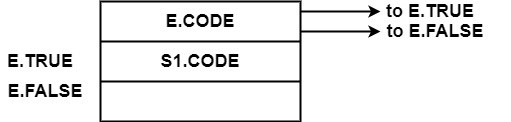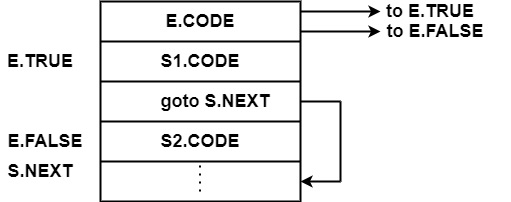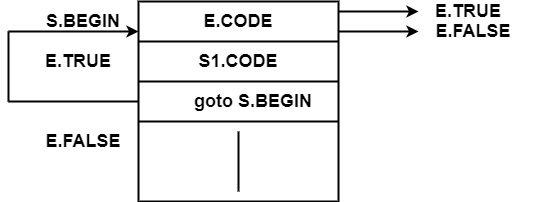# What is translation of control statements in compiler design?

Compiler DesignProgramming LanguagesComputer Programming

Control statements are the statements that change the flow of execution of statements.

Consider the Grammar

S → if E then S1

|if E then S1 else S2

|while E do S1

In this grammar, E is the Boolean expression depending upon which S1 or S2 will be executed.

Following representation shows the order of execution of an instruction of if-then, ifthen-else, & while do.

• 𝐒 → 𝐢𝐟 𝐄 𝐭𝐡𝐞𝐧 𝐒𝟏E.CODE & S.CODE are a sequence of statements which generate three address code.

E.TRUE is the label to which control flow if E is true.

E.FALSE is the label to which control flow if E is false.

∴ E.FALSE=S.NEXT in the following table.

In the following table, a new label is allocated to E.TRUE.

When S1.CODE will be executed, and the control will be jumped to statement following S, i.e., to S1.NEXT.

∴ S1. NEXT = S. NEXT.

Syntax Directed Translation for "If E then S1."

ProductionSemantic Rule
𝐒 → 𝐢𝐟 𝐄 𝐭𝐡𝐞𝐧 𝐒𝟏E. TRUE = newlabel;
E. FALSE = S. NEXT;
S1. NEXT = S. NEXT;
S. CODE = E. CODE | |
GEN (E. TRUE '− ') | | S1. CODE
• 𝐒 → 𝐈𝐟 𝐄 𝐭𝐡𝐞𝐧 𝐒𝟏 𝐞𝐥𝐬𝐞 𝐒𝟐If E is true, control will go to E.TRUE, i.e., S1.CODE will be executed and after that S.NEXT appears after S1.CODE.

If E.CODE will be false, then S2.CODE will be executed.

Initially, both E.TRUE & E.FALSE are taken as new labels. Hen S1.CODE at label E.TRUE is executed, control will jump to S.NEXT.

Therefore, after S1, control will jump to the next statement of complete statement S.

S1.NEXT=S.NEXT

Similarly, after S2.CODE, the next statement of S will be executed.

∴ S2.NEXT=S.NEXT

Syntax Directed Translation for "If E then S1 else S2."

ProductionSemantic Rule
𝐒 → 𝐢𝐟 𝐄 𝐭𝐡𝐞𝐧 𝐒𝟏 𝐞𝐥𝐬𝐞 𝐒𝟐E. TRUE = newlabel;
E. FALSE = newlabel;
S1. NEXT = S. NEXT;
S2. NEXT = S. NEXT;
S. CODE = E. CODE | | GEN (E. TRUE '− ') | | S1. CODE
GEN(goto S. NEXT) | |
GEN (E. FALSE −) | | S2. CODE
• 𝐒 → 𝐰𝐡𝐢𝐥𝐞 𝐄 𝐝𝐨 𝐒𝟏

Another important control statement is while E do S1, i.e., statement S1 will be executed till Expression E is true. Control will arrive out of the loop as the expression E will become false.A Label S. BEGIN is created which points to the first instruction for E. Label E. TRUE is attached with the first instruction for S1. If E is true, control will jump to the label E. TRUE & S1. CODE will be executed. If E is false, control will jump to E. FALSE. After S1. CODE, again control will jump to S. BEGIN, which will again check E. CODE for true or false.

∴ S1. NEXT = S. BEGIN

If E. CODE is false, control will jump to E. FALSE, which causes the next statement after S to be executed.

∴ E. FALSE = S. NEXT

Syntax Directed Translation for " 𝐒 → 𝐰𝐡𝐢𝐥𝐞 𝐄 𝐝𝐨 𝐒𝟏 "

ProductionSemantic Rule
𝐒 → 𝐰𝐡𝐢𝐥𝐞 𝐄 𝐝𝐨 𝐒𝟏S. BEGIN = newlabel;
E. TRUE = newlabel;
E. FALSE = S. NEXT;
S1. NEXT = S. BEGIN;
S. CODE = GEN(S. BEGIN '− ') | |
E. CODE | | GEN(E. TRUE '− ')| |
S1. CODE | | GEN('goto' S. BEGIN)